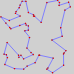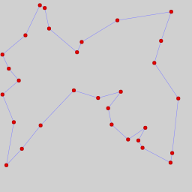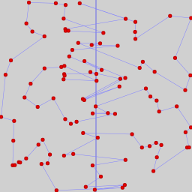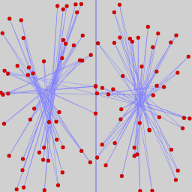# Simulated Annealing

## The Travelling Salesman ProblemYour browser does not support HTML5 canvas.
 cities Minimise Maximise path length   River cost Trace solution TSPLIB tools

## Travelling Salesman

The travelling salesman problem is one of the most-studied problems in combinatorial optimisation. It couldn't be easier to state:

Given a list of cities and their locations (usually specified as Cartesian co-ordinates on a plane), what is the shortest itinerary which will visit every city exactly once and return to the point of origin?

Easy to ask, but devilishly difficult to answer…. The obvious way to solve the travelling salesman problem would be to write down all of the possible sequences in which the cities could be visited, compute the distance of each path, and then choose the smallest. But the number of possible itineraries for visiting n cities grows as the factorial of n, which is written, appropriately, as “n!”. The factorial of a positive integer is the product of that number and all smaller numbers down to one. Hence 2!=2, 3!=6, 6!=720, and 10!=3,628,800. As you can see, these numbers grow very rapidly, so as you increase the number of cities, the number of paths you have to compare blows up in a combinatorial explosion which makes finding the optimal path by brute force computation a hopeless undertaking.

“But”, you ask, “computers are getting faster every year. Why not just be patient and wait a few years?” Neither you, nor I, nor the universe has sufficient patience. The box at the top of this page contains thirty cities represented by red balls placed at random in the grey square, connected by a path drawn in blue lines in the order in which they were placed. Every time you press the “Place” button, thirty new randomly-placed cities are generated; you can change the number by setting the box to the right of the button. But let's stick with thirty cities for the nonce.

The number of possible paths along which we can visit the thirty cities is equal to the number of permutations of a set of thirty distinct members, which is equal to the factorial of the number of members, or 30!. This is a breathtakingly large number.

30! = 265,252,859,812,191,058,636,308,480,000,000 ≈ 2.6525×1032

Now, let's assume you had a supercomputer which was able to compute the value of a billion (109) paths per second. Chugging away at this task around the clock, without a day of rest, it would take 2.65×1023 seconds to get through the list. How long is that? About 8.4 quadrillion (1015) years, or about 600,000 times the present age of the universe. And if you modestly increased the number of cities to fifty? Prepare to wait eight thousand billion billion times the age of the universe for the answer.Now scroll back up to the top of the page and click the “Solve” button Almost instantaneously, you'll see a near-optimal path to tour the thirty cities with the least distance of travel. Try clicking “Place” and then “Solve” several times to create and solve new problems, then increase the number of cities to 50 and then 100 and try solving those problems. In each case, the solution appears in a fraction of a second. Now, these solutions are not guaranteed to be absolutely optimal; they may be a percent or two longer than the absolute best path (if you click “Solve” multiple times, you may see several different solutions, all of which are close in total path length). They're not perfect, but then you don't have to wait huge multiples of the age of the universe for the result. How did we do it?

## Simulated Annealing

This page attacks the travelling salesman problem through a technique of combinatorial optimisation called simulated annealing. By analogy with the process of annealing a material such as metal or glass by raising it to a high temperature and then gradually reducing the temperature, allowing local regions of order to grow outward, increasing ductility and reducing stresses in the material, the algorithm randomly perturbs the original path to a decreasing extent according to a gradually decreasing logical “temperature”.

In simulated annealing, the equivalent of temperature is a measure of the randomness by which changes are made to the path, seeking to minimise it. When the temperature is high, larger random changes are made, avoiding the risk of becoming trapped in a local minimum (of which there are usually many in a typical travelling salesman problem), then homing in on a near-optimal minimum as the temperature falls. The temperature falls in a series of steps on an exponential decay schedule where, on each step, the temperature is 0.9 times that of the previous step.

The process of annealing starts with a path which simply lists all of the cities in the order their positions were randomly selected (this is the path you'll see after pressing the “Place” button). On each temperature step, a number of random transformations of the path are made. First of all, a segment of the path is selected, with its start and end cities chosen at random. Then, a software coin is flipped to decide which kind of transformation to try: reverse or transport.

If reverse comes up, an alternative path is generated in which the cities in the chosen segment are reversed in order of visit. If transport, the segment is clipped out of its current position in the path and spliced in at a randomly chosen point in the remainder of the path. The length of the modified path is then calculated and compared to the path before modification, producing a quantity called the cost difference. If negative, the modified path is shorter than the original path and always replaces it. If there is an increase in cost, however, the exponential of its negative magnitude divided by the current temperature is compared to a uniformly distributed random number between 0 and 1 and, if greater, the modified path will be used even though it increased the cost. Note that initially, when the temperature is high, there will be a greater probability of making such changes, but that as the temperature falls, only smaller increases in cost will be accepted. The total number of changes tested at each temperature level is arbitrarily set to 100 times the number of cities in the path, and after ten times the number of changes which decrease the path length as the number of cities are found, the temperature is decreased and the search continued. If, after trying all of the potential changes at a given temperature level, no changes are found which reduce the path length, the solution is considered “good enough” and the resulting path is displayed.

## Watching it Happen

To watch the optimisation process as it unfolds, instead of pressing the “Solve” button, press the “Step” button to see the path evolve at each level of decreasing temperature. The “Animate” button will automatically show the path evolving at one second per temperature level. Check the “Trace solution” box to display the temperature, cost (path length), and number of changes made to the path at each step in the optimisation. After a solution is found, the chosen itinerary will be shown listing the cities in order, their co-ordinates, and the cost of the path from each city to the next (wrapping around at the bottom) and, if the path crosses the river (see below), an “R” to indicate that it does.

Instead of using the “Place” button to randomly place cities, you can place them manually by pressing “New” to clear the map and then click the mouse in the map to indicate the city locations. They will initially be connected by paths in the order you placed the cities. You can also add cities to maps created by the “Place” button by clicking in the map.

## Minimise or Maximise?The travelling salesman problem is usually formulated in terms of minimising the path length to visit all of the cities, but the process of simulated annealing works just as well with a goal of maximising the length of the itinerary. If you change the goal in the drop-down list from “Minimise” to “Maximise”, the cost function being optimised will be the negative of the path length, resulting in a search for the longest path. Try it, and see how the annealing process finds a star-like pattern that chooses the longest inter-city paths.

## A River Runs through ItWe can add another wrinkle to the cost function by adding a “river” that runs through the map from top to bottom halfway across it. If you set the “River cost” nonzero, the river will be drawn as a dark blue line, and any path from one city to another which crosses it is assessed a penalty given by the river cost as a percentage of the size of the map. If you set the river cost high, say to 50%, you'll find a strong preference for paths which only cross the river twice, finding a near-minimum path length independently on each side of the river. (This may be more apparent if you place a large number of cities, say 100 or 250.)You can also set the cost of crossing the river negative, which turns the travelling salesman into a peripatetic smuggler who profits from carrying goods between Freedonia and Grand Fenwick. Try placing 100 cities and setting the river cost to −25: the smuggler will settle on an efficient path on each side of the river, but prefer river crossings between cities close to the river where the benefit of the crossing is significant compared to the distance between them.Finally, try setting the goal to Maximise path length, the river crossing cost to −100 (benefit from crossing the river), and place 100 cities. When you solve, you'll find the solution produces two star-like patterns on each side of the river which maximises the travel distance on each side, but avoids river crossings at all costs.

## Other Optimisation Techniques

Here we've explored one technique of combinatorial optimisation: simulated annealing. This is only one of the many approaches which have been taken to problems of this kind. These include exact approaches such as branch and bound, linear programming, and cutting-plane algorithms.

There are many approximation techniques which find near-optimal solutions, of which simulated annealing is but one. One algorithm even models how ants find the shortest path between their anthill and a source of food.

## Solving TSPLIB Problems

TSPLIB is a collection of problems of the travelling salesman type originally published by Gerhard Reinelt of the University of Heidelberg. The symmetric travelling salesman collection (the kind of problem discussed here) contains 111 problems ranging from 14 to 85900 “cities”, with data drawn from geography, printed and integrated circuit layout, and totally synthetic data sets. A total of 78 of these problems represent points in two-dimensional Euclidean space as used in this page, and can be solved by it. Of these problems, 19 have known optimal solutions which are included in TSPLIB.

You can experiment with these and other problems in TSPLIB format by checking the “TSPLIB tools” box. A control panel will appear below the trace solution box (if displayed) consisting of a multi-purpose text area and a series of controls.

Clear
Clears the text box.
Loads the problem in the text box, in TSPLIB format, into the solver. The problem should be defined with “EDGE_WEIGHT_TYPE : EUC_2D”. See one of the standard problems written in this format for an example of how they are defined.
Loads a TSPLIB optimum solution file from the text box. These files consist of a list of city numbers from a TSPLIB problem file in the optimimum solution order. See one of the TSPLIB “.opt.tour” files for an example of the format.
Save Problem
The current problem, regardless of its origin, is placed in the text box in TSPLIB format. Note that since TSPLIB problems are rescaled to a co-ordinate range of 0–1 when they are loaded, saving such a problem will not produce a file identical to that loaded.
Save Solution
The most-recently computed solution to the current problem is written to the text box in TSPLIB “.opt.tour” format. Note that since the solutions we compute are approximate, a solution found by this page should not be presented as optimal nor saved with a file name implying it is.
Problem
Loads a problem or solution in TSPLIB format from a file on your local computer by selecting the file from the “Choose File” box (problem files have a file type of “.tsp” while solution files end with “.tour”). The problem or solution file is loaded into the text box and the solver when you press Load. If you are loading a problem and solution, the problem must be loaded first.
Problem URL
Loads a problem or solution in TSPLIB format from the specified URL on the Web. (Some browsers may restrict the sites from which you can load URLs due to protections against “cross-site scripting”; this is beyond the control of this page.) The URL should specify a file with a file type of “.tsp” for problems and “.tour”) for solutions. The problem or solution file is loaded into the text box and the solver when you press Load. If you are loading a problem and solution, the problem must be loaded first.

Below the URL box, a drop-down list allows you to select 78 problems from TSPLIB which are available on the Fourmilab server. Those shown with a green background have known optimal solutions, which will be loaded automatically when the problem is loaded.
Show optimal solution
If a solution file has been loaded, its paths will be shown in green in the map window if this box is checked. If no solution has been loaded, the check box will be disabled. Where optimal paths and those found by the solver agree, they will be drawn in green: those found by the solver which differ from the optimum are shown in blue. Note that the paths shown in green are only optimal when the solution file loaded has been proved to be optimal; this is the case for all of those loaded from the TSPLIB drop-down box.

## Reference

Press, William H., Saul A. Teukolsky, William T. Vetterling, and Brian P. Flannery. Numerical Recipes in C, 2nd ed. Cambridge: Cambridge University Press,  1992. ISBN 978-0-521-43108-8. Section 10.9, pp. 444–451. (A third edition of this book with algorithms in C++ is available.)

by John Walker
June, 2018